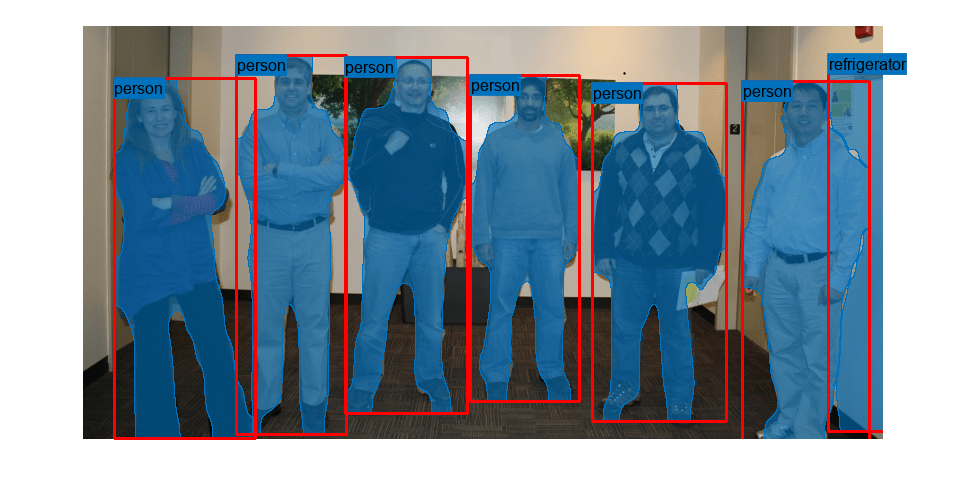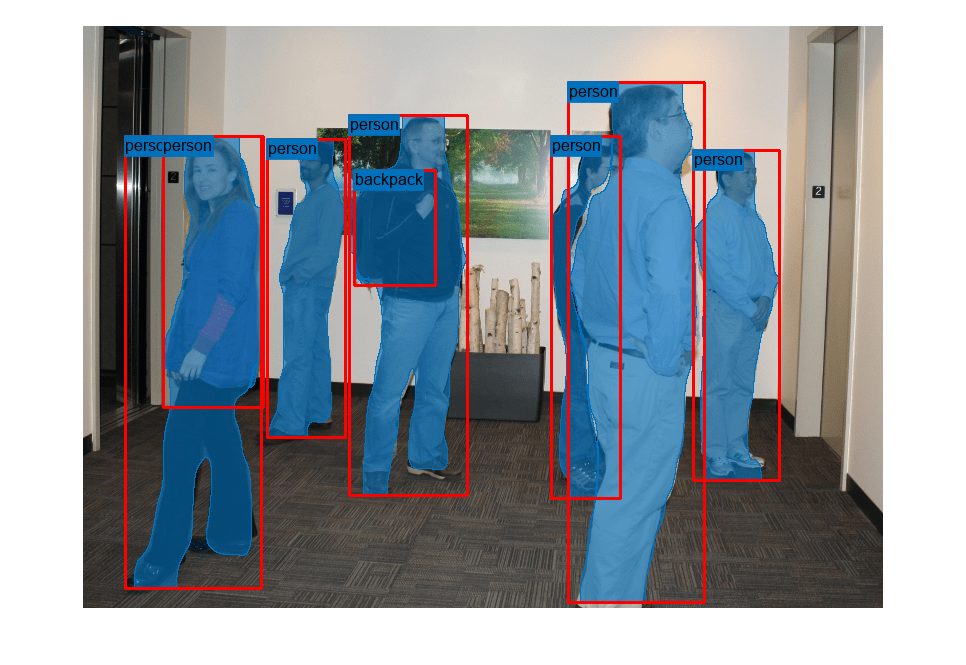# segmentObjects

Segment objects using Mask R-CNN instance segmentation

Since R2021b

## Syntax

``masks = segmentObjects(detector,I)``
``[masks,labels] = segmentObjects(detector,I)``
``[masks,labels,scores] = segmentObjects(detector,I)``
``[masks,labels,scores,bboxes] = segmentObjects(detector,I)``
``dsResults = segmentObjects(detector,imds)``
``[___] = segmentObjects(___,Name=Value)``

## Description

````masks = segmentObjects(detector,I)` detects object masks within a single image or an array of images, `I`, using a Mask R-CNN object detector.```
````[masks,labels] = segmentObjects(detector,I)` also returns the labels assigned to the detected objects.```
````[masks,labels,scores] = segmentObjects(detector,I)` also returns the detection score for each of the detected objects. ```

example

````[masks,labels,scores,bboxes] = segmentObjects(detector,I)` also returns the location of segmented object as bounding boxes, `bboxes`.```

example

````dsResults = segmentObjects(detector,imds)` performs instance segmentation of images in a datastore using a Mask R-CNN object detector. The function returns a datastore with the instance segmentation results, including the instance masks, labels, detection scores, and bounding boxes.```
````[___] = segmentObjects(___,Name=Value)` configures the segmentation using additional name-value arguments. For example, `segmentObjects(detector,I,Threshold=0.9)` specifies the detection threshold as `0.9`. NoteThis function requires the Computer Vision Toolbox™ Model for Mask R-CNN Instance Segmentation. You can install the Computer Vision Toolbox Model for Mask R-CNN Instance Segmentation from Add-On Explorer. For more information about installing add-ons, see Get and Manage Add-Ons. To run this function, you will require the Deep Learning Toolbox™. ```

## Examples

collapse all

`detector = maskrcnn("resnet50-coco")`
```detector = maskrcnn with properties: ModelName: 'maskrcnn' ClassNames: {1×80 cell} InputSize: [800 1200 3] AnchorBoxes: [15×2 double] ```

Read a test image that includes objects that the network can detect, such as people.

`I = imread("visionteam.jpg");`

Segment instances of objects using the Mask R-CNN object detector.

`[masks,labels,scores,boxes] = segmentObjects(detector,I,Threshold=0.95);`

Overlay the detected object masks in blue on the test image. Display the bounding boxes in red and the object labels.

```overlayedImage = insertObjectMask(I,masks); imshow(overlayedImage) showShape("rectangle",boxes,Label=labels,LineColor=[1 0 0])````detector = maskrcnn("resnet50-coco");`

Create a datastore of test images.

```imageFiles = fullfile(toolboxdir("vision"),"visiondata","visionteam*.jpg"); dsTest = imageDatastore(imageFiles);```

Segment instances of objects using the Mask R-CNN object detector.

`dsResults = segmentObjects(detector,dsTest);`
```Running Mask R-CNN network -------------------------- * Processed 2 images. ```

For each test image, display the instance segmentation results. Overlay the detected object masks in blue on the test image. Display the bounding boxes in red and the object labels.

```while(hasdata(dsResults)) testImage = read(dsTest); results = read(dsResults); overlayedImage = insertObjectMask(testImage,results{1}); figure imshow(overlayedImage) showShape("rectangle",results{4},Label=results{2},LineColor=[1 0 0]) end```## Input Arguments

collapse all

Mask R-CNN object detector, specified as a `maskrcnn` object.

Image or batch of images to segment, specified as one of these values.

Image TypeData Format
Single grayscale image2-D matrix of size H-by-W
Single color image3-D array of size H-by-W-by-3.
Batch of B grayscale or color images4-D array of size H-by-W-by-C-by-B. The number of color channels C is 1 for grayscale images and 3 for color images.

The height H and width W of each image must be greater than or equal to the input height h and width w of the network.

Datastore of images, specified as a datastore such as an `imageDatastore` or a `CombinedDatastore`. If calling the datastore with the `read` function returns a cell array, then the image data must be in the first cell.

### Name-Value Arguments

Specify optional pairs of arguments as `Name1=Value1,...,NameN=ValueN`, where `Name` is the argument name and `Value` is the corresponding value. Name-value arguments must appear after other arguments, but the order of the pairs does not matter.

Example: `segmentObjects(detector,I,Threshold=0.9)` specifies the detection threshold as `0.9`.

Options for All Image Formats

collapse all

Detection threshold, specified as a numeric scalar in the range [0, 1]. The Mask R-CNN object detector does not return detections with scores less than the threshold value. Increase this value to reduce false positives.

Maximum number of strongest region proposals, specified as a positive integer. Reduce this value to speed up processing time at the cost of detection accuracy. To use all region proposals, specify this value as `Inf`.

Select the strongest bounding box for each detected object, specified as a numeric or logical `1` (`true`) or `0` (`false`).

• `true` — Return the strongest bounding box per object. To select these boxes, the `segmentObjects` function calls the `selectStrongestBboxMulticlass` function, which uses nonmaximal suppression to eliminate overlapping bounding boxes based on their confidence scores.

• `false` — Return all detected bounding boxes. You can then create your own custom operation to eliminate overlapping bounding boxes.

Minimum size of a region containing an object, in pixels, specified as a two-element numeric vector of the form [height width]. By default, `MinSize` is the smallest object that the trained detector can detect. Specify this argument to reduce the computation time.

Maximum size of a region containing an object, in pixels, specified as a two-element numeric vector of the form [height width].

To reduce computation time, set this value to the known maximum region size for the objects being detected in the image. By default, `MaxSize` is set to the height and width of the input image, `I`.

Hardware resource for processing images with a network, specified as `"auto"`, `"gpu"`, or `"cpu"`.

`ExecutionEnvironment`Description
`"auto"`Use a GPU if available. Otherwise, use the CPU. The use of GPU requires Parallel Computing Toolbox™ and a CUDA® enabled NVIDIA® GPU. For information about the supported compute capabilities, see GPU Computing Requirements (Parallel Computing Toolbox).
`"gpu"`Use the GPU. If a suitable GPU is not available, the function returns an error message.
`"cpu"`Use the CPU.

Options for Datastore Inputs

collapse all

Number of observations that are returned in each batch. The default value is equal to the `ReadSize` property of datastore `imds`.

You can specify this argument only when you specify a datastore of images, `imds`.

Location to place writable data, specified as a string scalar or character vector. The specified folder must have write permissions. If the folder already exists, the `segmentObjects` function creates a new folder and adds a suffix to the folder name with the next available number. The default write location is `fullfile(pwd,"SegmentObjectResults")` where `pwd` is the current working directory.

You can specify this argument only when you specify a datastore of images, `imds`.

Data Types: `char` | `string`

Prefix added to written filenames, specified as a string scalar or character vector. The files are named `<NamePrefix>_<imageName>.mat`, where `imageName` is the name of the input image without its extension.

You can specify this argument only when you specify a datastore of images, `imds`.

Data Types: `char` | `string`

Enable progress display to screen, specified as a numeric or logical `1` (`true`) or `0` (`false`).

You can specify this argument only when you specify a datastore of images, `imds`.

## Output Arguments

collapse all

Objects masks, returned as a logical array of size H-by-W-by-M. H and W are the height and width of the input image `I`. M is the number of objects detected in the image. Each of the M channels contains the mask for a single detected object.

When `I` represents a batch of B images, `masks` is returned as a B-by-1 cell array. Each element in the cell array indicates the masks for the corresponding input image in the batch.

Objects labels, returned as an M-by-1 categorical vector where M is the number of detected objects in image `I`.

When `I` represents a batch of B images, then `labels` is a B-by-1 cell array. Each element is an M-by-1 categorical vector with the labels of the objects in the corresponding image.

Detection confidence scores, returned as an M-by-1 numeric vector, where M is the number of detected objects in image `I`. A higher score indicates higher confidence in the detection.

When `I` represents a batch of B images, then `scores` is a B-by-1 cell array. Each element is an M-by-1 numeric vector with the labels of the objects in the corresponding image.

Location of detected objects within the input image, returned as an M-by-4 matrix, where M is the number of detected objects in image `I`. Each row of `bboxes` contains a four-element vector of the form [x y width height]. This vector specifies the upper left corner and size of that corresponding bounding box in pixels.

When `I` represents a batch of B images, then `bboxes` is a B-by-1 cell array. Each element is an M-by-4 numeric matrix with the bounding boxes of the objects in the corresponding image.

Predicted instance segmentation results, returned as a `FileDatastore` object. The datastore is set up so that calling the datastore with the `read` and `readall` functions returns a cell array with four columns. This table describes the format of each column.

RGB image that serves as a network input, returned as an H-by-W-by-3 numeric array.

Bounding boxes, returned as M-by-4 matrices, where M is the number of objects within the image. Each bounding box has the format [x y width height], where [x, y] represent the top-left coordinates of the bounding box.

Object class names, returned as an M-by-1 categorical vector. All categorical data returned by the datastore must contain the same categories.

Binary masks, returned as a logical array of size H-by-W-by-M. Each mask is the segmentation of one instance in the image.

## Version History

Introduced in R2021b

expand all